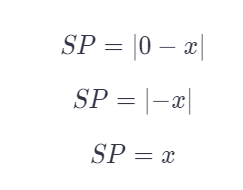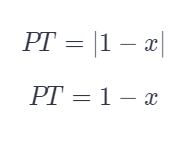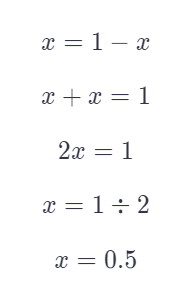# Points S, T, and P lie on a number line. Their coordinates are 0, 1, and x, respectively.

We thoroughly check each answer to a question to provide you with the most correct answers. Found a mistake? Let us know about it through the REPORT button at the bottom of the page.

Points S, T, and P lie on a number line. Their coordinates are 0, 1, and x, respectively. Given SP = PT, what is the value of x?

## Explanation

Use the coordinates of points S and P and find the length of SP. Since the points are on a number line, the length of the segment is given by the absolute value of the difference of the coordinates of the points.Use the coordinates of points P and T and find the length of PT. Since the points are on a number line, the length of the segment is given by the absolute value of the difference of the coordinates of the points.Since SP and PT have equal lengths, you can use the expressions for their lengths, set them equal, and solve the equation that you get for x.